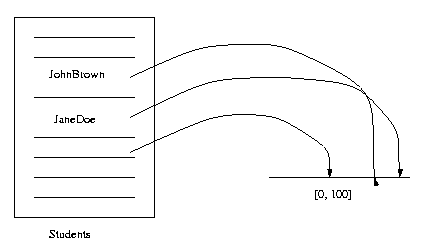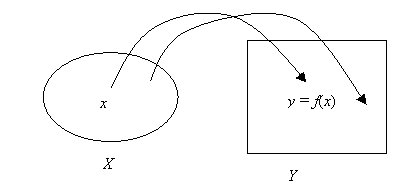EECS20N: Signals and Systems

Functions on sets

A function or mapping is a relationship between one set (called the domain) and another set (called the range). To each element of the domain is assigned an element of the range. For example:
• The function Score assigns a test scores to each member of the set Students = {JohnBrown, JaneDoe, JamesChen, ...}.
• The domain of the function Score is Students and the range is Scores = [0, 100].
We also use the notations:
• Score: Students → [0, 100]
• Score(JohnBrown) = 90, Score(JaneDoe) = 90.2, ...In general, a function is a triple f : X Y, where X and Y are sets: X is the domain of f, Y is the range of f, and f is the name of a rule that assigns the value f(x) in Y to each element x in X.

If x is a variable over X, we also say f is a function of x. For each element x in the domain, we write f(x) for the element of the range that is assigned to that value x.